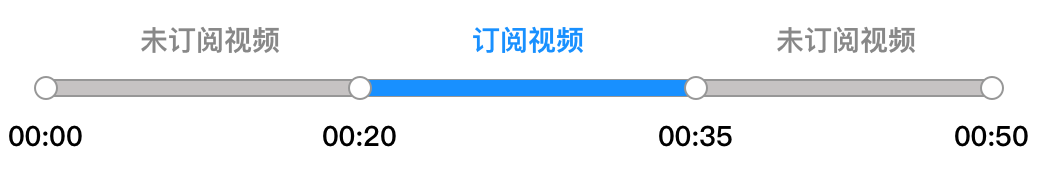# 音视频时长计费说明

## 注意事项

• 计费方式：日结后付费计费。
• 计费周期：按日结算，每日音视频时长费用将于次日出计费账单时扣除，详细计费和出账时间以实际 计费账单 为准。
• 支持购买 预付费套餐包，默认采用预付费套餐包，当服务用量无套餐包可抵扣或超出套餐包余量时，将采用后付费计费。
• 免费试用套餐包可用于抵扣音视频时长服务用量，详情请参见 免费试用
• 您可以使用 TRTC 价格计算器 预估自己业务将产生的音视频时长用量和费用。
• TRTC 全平台 SDK 都不会限制您的视频分辨率，您可以根据实际业务需求自行 设定画面质量
• 若将 TRTC 房间内的音频流旁路到云直播系统中，让观众通过直播 CDN 观看，将产生额外的费用，详情请参见 CDN 直播观看 > 相关费用

## 计费价格

2K 1920x1080-2560 x1440（含） 112.00
4K 2560x1440-4096 x 2176（含） 252.00

• 按分辨率面积计算分辨率档位。如，720×360的分辨率，面积小于640×480，所以在标清档位内。
• 订阅指用户向 TRTC 服务端请求拉取指定用户音视频数据的操作，对应“拉流”。

## 用量统计

### 视频时长

• 视频时长定义为：订阅视频流的时长（接收到视频画面的时长）。
• 用户可能会在同一个房间内多次进出，TRTC 会实时统计其多段视频时长后叠加计算。
• 单个用户同时订阅多路视频时，其订阅的每一路视频时长将分别统计后叠加计算。
• TRTC 会根据用户实际接收到的视频分辨率划分视频档位，然后分别对不同档位的视频时长进行计费。

### 音频时长

• 音频时长定义为：未订阅视频流时，即产生音频时长（包括：仅订阅音频、仅在房、在房仅向房间内发送音视频数据）。
• 房间内仅有一个用户时，按音频时长统计其在房费用，音频时长为该用户在房总时长。
• 用户可能会在同一个房间内多次进出，TRTC 会实时统计其多段音频时长后叠加计算。

## 计费示例

### 用量示例``音频时长=在房时长 - 订阅视频的时长 = 50分钟 - 15分钟 = 35分钟``

``视频时长=订阅视频时长 = 15分钟 ``

### 纯音频计费示例

• 纯音频时长计费 = 音频时长单价 × 所有用户在房时长之和
• 则该 TRTC 房间产生的音频时长总费用为：音频时长单价 × 所有用户音频时长之和 = 7.00元/千分钟 ×（30分钟 + 30分钟 + 30分钟） / 1000 = 0.63元。

### 纯视频计费示例

A
640 × 360（标清）
B
1920 × 1080（超高清）

A - 发送视频，订阅 B 的视频
B - 发送视频，订阅 A 的视频

• A 产生的用量及费用：A 产生的费用 = A 接收 B 的费用 = 超高清视频时长单价 × 超高清视频时长 = 63元/千分钟 × (30分钟 / 1000) = 1.89 元
• B 产生的用量及费用：B 产生的费用 = B 接收 A 的费用 = 标清视频时长单价 × 标清时长 = 14元/千分钟 × (30分钟 / 1000) = 0.42 元

### 语音/视频混合计费示例

A
640 × 360（标清）
B

C
1920 × 1080（超高清）

A - 发送视频，订阅 B 的音频，订阅 C 的视频
B - 发送音频，订阅 A 的视频，订阅 C 的视频
C - 发送视频，订阅 A 的视频，订阅 B 的音频

• A 产生的用量及费用：A 产生的费用 = A 接收 B 的费用 + A 接收 C 的费用
• A 接收 B 的费用 = 语音时长单价 × 语音时长 = 7元/千分钟 × (30分钟 / 1000）= 0.21 元
• A 接收 C 的费用 = 超高清视频时长单价 × 超高清视频时长 = 63元/千分钟 × (30分钟 / 1000) = 1.89 元
• A 产生的费用为 A 接收 B 的费用 + A 接收 C 的费用 = 0.21 + 1.89 = 2.10元
• B 产生的用量及费用：B 产生的费用 = B 接收 A 的费用 + B 接收 C 的费用
• B 接收 A 的费用 = 标清视频时长单价 × 标清时长 = 14元/千分钟 × (30分钟 / 1000) = 0.42 元
• B 接收 C 的费用 = 超高清时长单价 × 超高清时长 = 63元/千分钟 × (30分钟 / 1000）= 1.89 元
• B 产生的费用为 = B 接收 A 的费用 + B 接收 C 的费用 = 0.42 + 1.89 = 2.31 元
• C 产生的用量及费用：C 产生的费用 = C 接收 A 的费用 + C 接收 B 的费用
• C 接收 A 的费用 = 标清视频时长单价 × 标清时长 = 14元/千分钟 × (30分钟 / 1000) =0.42 元
• C 接收 B 的费用 = 语音时长单价 × 语音时长 = 7元/千分钟 × (30分钟 / 1000）= 0.21 元
• C 产生的费用为 = C 接收 A 的费用 + C 接收 B 的费用 = 0.42 + 0.21 =0.63元